# Stanley-Reisner ring

(diff) ← Older revision | Latest revision (diff) | Newer revision → (diff)
Jump to: navigation, search

Stanley–Reisner face ring, face ring

The Stanley–Reisner ring of a simplicial complexover a fieldis the quotient ring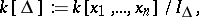where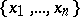are the vertices of,denotes the polynomial ring overin the variables, andis the ideal in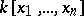generated by the non-faces of, i.e.,The support of any monomial inis a face of. In particular, the square-free monomials of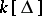correspond bijectively to the faces of, and are therefore called the face-monomials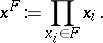One may thus writemore compactly as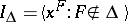.

It is easy to verify that the Krull dimension of(cf. also Dimension) is one greater than the dimension of(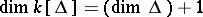).

Recall that the Hilbert series of a finitely-generated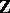-graded moduleover a finitely-generated-algebra is defined by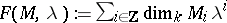. The Hilbert series ofmay be described from the combinatorics of. Let, let, and call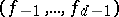the-vector of. Thenwhere the sequence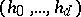, called the-vector of, may be derived from the-vector of(and vice versa) by the equation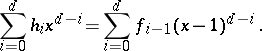The mapping fromtoallows properties defined for rings to be naturally extended to simplicial complexes. The most well-known and useful example is Cohen–Macaulayness: A simplicial complexis defined to be Cohen–Macaulay (over the field) whenis Cohen–Macaulay (cf. also Cohen–Macaulay ring). The utility of this extension is demonstrated in the proof that if (the geometric realization of) a simplicial complex is homeomorphic to a sphere, then its-vector satisfies a condition called the upper bound conjecture (for details, see [a1], Sect. II.3,4). The statement of this result requires no algebra, but the proof relies heavily upon the Stanley–Reisner ring and Cohen–Macaulayness. Many other applications of the Stanley–Reisner ring may be found in [a1], Chaps. II, III.

Finally, there is an anti-commutative version of the Stanley–Reisner ring, called the exterior face ring or indicator algebra, in which the polynomial ringin the definition ofis replaced by the exterior algebra.

How to Cite This Entry:
Stanley-Reisner ring. Encyclopedia of Mathematics. URL: http://encyclopediaofmath.org/index.php?title=Stanley-Reisner_ring&oldid=11800
This article was adapted from an original article by Art Duval (originator), which appeared in Encyclopedia of Mathematics - ISBN 1402006098. See original article